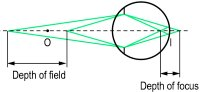depth of focus

(redirected from depths of focus)
Also found in: Dictionary, Encyclopedia.

fo·cal depth

, depth of focus
the greatest distance through which an object point can be moved while maintaining a clear image.
Synonym(s): penetration (3)

fo·cal depth

, depth of focus (fō'kăl depth, fō'kŭs)
The greatest distance through which an object point can be moved while remaining in focus.

depth of focus

For a given setting of an optical system (or a steady state of accommodation of the eye) it is the distance in front and behind the focal point (or retina) over which the image may be focused without causing a sharpness reduction beyond a certain tolerable amount. (The criterion could be as much as a line of letters on a Snellen chart.) The depth of focus D is represented by the total distance in front and behind (Fig. D1). As with depth of field, it is inversely proportional to the diameter of the diaphragm (or pupil). It can be calculated, expressed in dioptres, using the equation
D = (2b/p)Fe
where Fe is the power of the emmetropic eye, p the pupillary diameter and b the maximum size of the retinal image beyond which it is perceived as blurred. Example: Assuming a pupil of 3 mm and a retinal image spread over five cones, each 0.002 mm in diameter and spaces between the cones of 0.0005 mm, that is a total size = 0.012 mm, D = 2 ✕ 0.012/3 ✕ 60 = 0.48 D. Thus an object appears clear if the vergence at the eye varies in the range ±0.24 D. If the eye is focused at infinity, the equation becomes D = (b/p) Fe.Fig. D1 Schematic representation of the depth of field and the depth of focus of an eye fixating an object at O (I, retinal image size corresponding to the tolerable resolution)

fo·cal depth

, depth of focus (fō'kăl depth, fō'kŭs)
The greatest distance through which a point can move in focus.
Site: Follow: Share:
Open / Close